Engineering ToolBox - Resources, Tools and Basic Information for Engineering and Design of Technical Applications!

# Levers

## Use levers to magnify forces.

A lever is a mechanism that can be used to exert a large force over a small distance at one end of the lever by exerting a small force over a greater distance at the other end.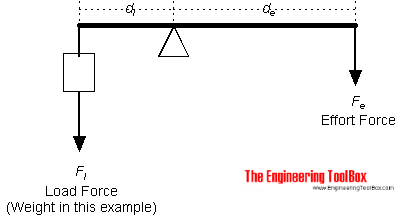The moment action on both sides of the lever is equal and can be expressed as

Fe de = Fl dl                             (1)

where

Fe = effort force (N, lb)

Fl = load force (N, lb) (note that weight is a force)

dl = distance from load force to fulcrum (m, ft)

de = distance from effort force to fulcrum (m, ft)

The effort force can be calculated by modifying (1) to

Fe = Fl dl / de

= m ag dl / de                        (1b)

where

m = mass (kg, slugs)

ag = acceleration of gravity (9.81 m/s2, 32.17 ft/s2)

### Lever Calculator

This calculator can used to calculate lever effort force. It can be used for both metric and imperial units as long as the use of units are consistent.

Fl - load force (N, kg, lb)

dl - distance form load force to fulcrum (m, ft)

de - distance from effort force to fulcrum (m, ft)

### Example - Improvised Car Lift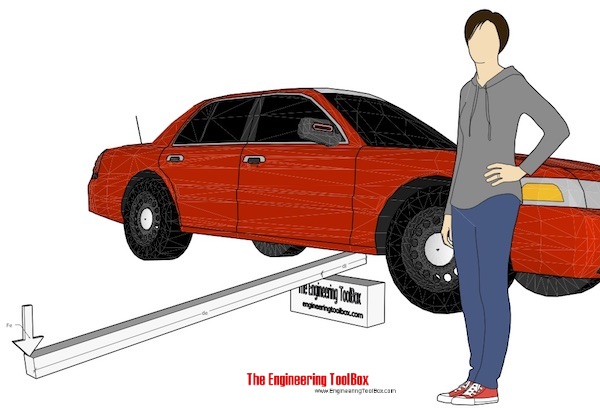An improvised car lift can be made with a piece of lumber as illustrated in the figure above. With distance load to fulcrum 0.2 m, distance effort force to fulcrum 2 m and a load on the lever - half the weight of the car 2000 kg - the effort force can be calculated

Fe = (0.5 2000 kg) (9.81 m/s2) (0.2 m) / (2 m)

= 981 N

≈ 100 kg

### Orders of Levers

#### First-order levers

• the fulcrum is positioned between the effort and the load
• the effort is smaller than the load
• the effort moves further than the load
• the lever can be considered as a force magnifier

#### Second-order Levers

• the effort and the load are positioned on the same side of the fulcrum but applied in opposite directions
• the load lies between the effort and the fulcrum
• the effort is smaller than the load
• the effort moves further than the load
• the lever can be considered as a force magnifier

#### Third-order Levers

• the effort lies between the load and the fulcrum
• the effort is greater than the load
• the load moves further than the effort
• the lever can be considered as a distance magnifier

### Example - First-Class (Order) Lever - A force (weight) of 1 pound is exerted at the end of a lever at distance 1 ft from the fulcrum

The effort force at a distance of 2 ft from the fulcrum can be calculated as

Fe = (1 lb) (1 ft) / (2 ft)

= 0.5 (lb)

The formula (1) can be modified to express required load if you know the effort, or required distance from fulcrum if load and effort forces are known and so on.

The level above where the fulcrum located between the load and effort force is often characterized as a first-class level mechanism.

A level where the load and effort force are located on the same side of the fulcrum is often characterized as a second-class level mechanism.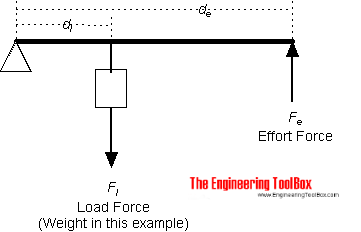### Example - Second-Class (Order) Lever

A force (weight) of 1 pound is exerted at a distance of 1 ft from the fulcrum.

The effort force at a distance of 2 ft from the fulcrum can be calculated as

Fe = (1 lb) (1 (ft) / (2 ft)

= 0.5 (lb)

### Example - Lever calculation with SI-units - weight of 1 kg mass acting 1 m from the fulcrum

The effort force at a distance of 2 m from the fulcrum can be calculated as

Fe = (1 kg) (9.81 m/s2) (1 m) / (2 m)

= 4.9 N

A lever mechanism where the input effort is higher than than the output load is often characterized as a third-class lever mechanism.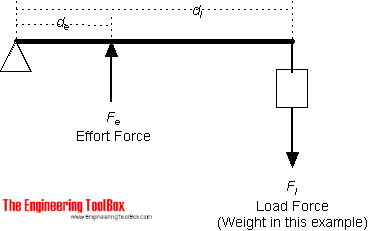### Example - Third-Class (Order) Lever

A force (weight) of 1 pound is exerted at a distance of 2 ft from the fulcrum.

The effort force at a distance of 1 ft from the fulcrum can be calculated as

Fe = Fl dl / de

= (1 lb) (2 ft) / (1 ft)

= 2 (lb)

### One or more forces acting on a lever

A lever with two acting load forces and one effort force is indicated in the sketch below: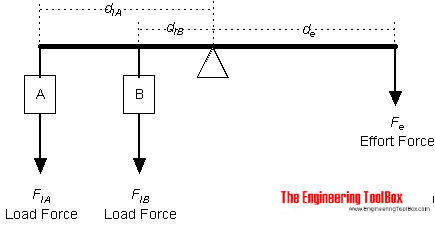The generic equation for one effort force with one or more acting load forces can be expressed as

Fe = (FlA dlA +  FlB dlB + .. + FlN dlN ) / de                                                (2)

This equation is modified for three acting loads below.

### Example - A Lever with three acting loads and one effort force

A weight A of 1 pound is exerted at a distance of 1 ft from the fulcrum. A weight B of 2 pound is exerted at a distance of 2 ft from the fulcrum, and a weight C of 3 pound is exerted at a distance of 3 ft from the fulcrum.

The effort force at a distance of 2 ft from the fulcrum can be calculated as

Fe = (FlA dlA +  FlB dlB + FlC dlC ) / de

= ((1 lb) (1 ft) + (2 lb) (2 ft) + (3 lb) (3 ft)) / (2 ft)

= 7 (lb)

## Related Topics

• Mechanics - Forces, acceleration, displacement, vectors, motion, momentum, energy of objects and more.
• Statics - Loads - forces and torque, beams and columns.

## Engineering ToolBox - SketchUp Extension - Online 3D modeling!

Add standard and customized parametric components - like flange beams, lumbers, piping, stairs and more - to your Sketchup model with the Engineering ToolBox - SketchUp Extension - enabled for use with the amazing, fun and free SketchUp Make and SketchUp Pro .Add the Engineering ToolBox extension to your SketchUp from the SketchUp Pro Sketchup Extension Warehouse!

Translate

## Privacy

We don't collect information from our users. Only emails and answers are saved in our archive. Cookies are only used in the browser to improve user experience.

Some of our calculators and applications let you save application data to your local computer. These applications will - due to browser restrictions - send data between your browser and our server. We don't save this data.

## Citation

• Engineering ToolBox, (2008). Levers. [online] Available at: https://www.engineeringtoolbox.com/levers-d_1304.html [Accessed Day Mo. Year].

Modify access date.

. .

#### Scientific Online Calculator6 24